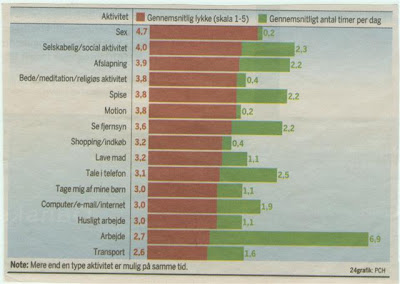# Another blue blog

## happiness scale

Here is something that i found in the local newspaper! :)Take a look at this! ;) I know that the tekst is on danish, so i will now translate it for you! :D

1. Sex gets 4,7 out of 5. The average number of hours we do this, per day. Is 0,2
2. Social activity gets 4 out of 5. The average number of hours we do this, per day. Is 2,3
3. Relaxing gets 3,9 out of 5. The average number of hours we do this, per day. Is 2,2
4. Religious activity gets 3,8 out of 5. The average number of hours we do this, per day. Is 0,4
5. Eating gets 3,8 out of 5. The average number of hours we do this, per day. Is 2,2
6. exercise gets 3,8 out of 5. The average number of hours we do this, per day. Is 0,2
7. Watching television gets 3,6 out of 5. The average number of hours we do this, per day. Is 2,2
8. Shopping gets 3,2 out of 5. The average number of hours we do this, per day. Is 0,4
9. Making dinner gets 3,2 out of 5. The average number of hours we do this, per day. Is 1,1
10. Talking in phone gets 3,1 out of 5. The average number of hours we do this, per day. Is 2,5
11. Taking care of my kids gets 3,0 out of 5. The average number of hours we do this, per day. Is 1,1
12. Computer, Email and Internet gets 3,0 out of 5. The average number of hours we do this, per day. Is 1,9
13. Work at home gets 3,0 out of 5. The average number of hours we do this, per day. Is 1,1
14. Work gets 2,7 out of 5. The average number of hours we do this, per day. Is 6,9
15. Transportation gets 2,6 out of 5. The average number of hours we do this, per day. Is 1,6

And what can we use these fact's to?

The average time spent by others, must be the time you need to spent at the different activities, to get that most out of them (Happiness). So these fact's can be used to help you and others, find out what they need to spend more time doing, or what they need to stop doing as much as they do.

The end

I hope that you liked my fact's about our everyday life. :) And i hope that, if you need help finding yourself in your life? That this will help you on your way.

Greeting from Jim! The owner of this blog.

#### 0 kommentarer: